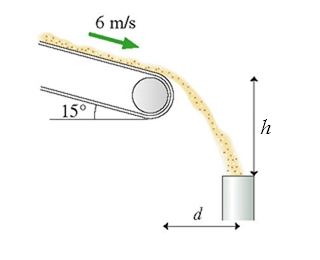# Problem: Sand moves without slipping at 6.0 m/s down a conveyer that is tilted at 15°. The sand enters a pipe  h = 3.6 m below the end of the conveyer belt, as shown in the figure.  What is the horizontal distance  d between the conveyer belt and the pipe?

###### FREE Expert Solution

This problem is asking us to find the horizontal distance traveled by the grains of sand given the magnitude and direction of the initial velocity and the height they fall.

For projectile motion problems in general, we'll follow these steps to solve:

1. Identify the target variable and known variables for each direction—remember that only 3 of the 5 variablesx or Δy, v0, vf, a, and t) are needed for each direction. Also, it always helps to sketch out the problem and label all your known information!
2. Choose a UAM equation—sometimes you'll be able to go directly for the target variable, sometimes another step will be needed in between.
3. Solve the equation for the target (or intermediate) variable, then substitute known values and calculate the answer.

The four UAM (kinematics) equations are:

We define our coordinate system so that the +y-axis is pointing upwards and the +x-direction is horizontal along the launch direction. That means ay = −g, and ax = 0 (because the only acceleration acting on a projectile once it's launched is gravity.)

For projectiles with a negative launch angle, we also need to know how to decompose a velocity vector into its x- and y-components:

83% (71 ratings)###### Problem Details

Sand moves without slipping at 6.0 m/s down a conveyer that is tilted at 15°. The sand enters a pipe  h = 3.6 m below the end of the conveyer belt, as shown in the figure.  What is the horizontal distance  d between the conveyer belt and the pipe?